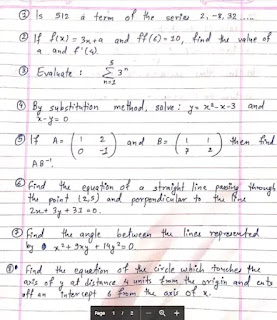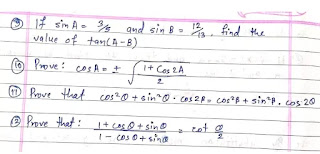# SEE model questions of optional math with answers 2076 | OPT Math class 10 question

0
In this blog I'll give you model question of class 10 optional math in Nepal in pdf format. You can download the see model questions of optional math with answers from the following links.

"These questions below are previously asked questions in SEE exam, So make sure you practice all the questions below"

After solving these questions comeback again to see the answers in the another pdf but before that don't download the another pdf.

FIRST SOLVE ALL THE QUESTIONS YOURSELF, AFTER COMEBACK TO CHECK THE ANSWER.

## 12 model questions of opt math for SEE

All these questions below are based on new syllabus of optional math class 10 in Nepal according to NEB government. Questions below are from the following chapters:

### Chapters from the Optional Math:

1. Algebra
2. Function
3. Polynomials
4. Geometric Sequence
5. Linear Programming
6. Matrix
7. Coordinate Geometry
8. Trigonometrymodel questions of optional mathoptional math model question class 10

1) How to remember all the formula of Opt math?
Look, it's difficult to remember all the formula but at the same time we can remember all the formulas chapter wise by making pneumonic. I used to make pneumonic of all formulas like by giving name of our friends to the formula or by writing the short form of each formula.

2) How exactly we can remember the formula of Opt math?
• by making it short and simple
• by making pneumonic
• by attaching in front of your study table
• by making it more familiar

3) How can I get full marks in Opt math?
You have to follow all the tips and tricks below to get full marks in Opt math:
• make sure you practice a lot
• make a proper environment of study
• It's not about how many hours you practice, its about how effectively you practiced in that hours.
•  Also watch this video to get good marks in Opt math
4) How to study trigonometry easily?
Haha, there is no short trick for trigonometry but here is how you can make it easier
• Don't study the out theorem of trigonometry
• Don't even touch the out theorem
• Practice previous year questions
• Make formula chart
• Attach that formula chart in front of your study table
• Try to understand the formula
• Revise, Revise and just Revise
So these are some frequently asked questions regarding Opt math preparations. For more detailed explanation, Watch the following Video:

Also you can subscribe to our YouTube Channel for Educational videos.

Also you can follow me on Instagram if you want to ask any questions.

Instagram Id = Ganesh Gautam

Also comment down what you want in next blog.
Tags